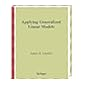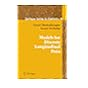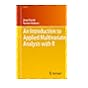Dr. S. R. Lasker Library Online Catalogue

Normal view

# Discovering structural equation modeling using Stata / Alan C Acock.

Material type:TextLanguage: English Publication details: 2013. Edition: Rev. edDescription: xxv, 306 p. : ill. ; 24 cmISBN: 9781597181396; 1597181331; 9781597181334Subject(s): Stata | Structural equation modelingDDC classification: 519.535 Online resources: WorldCat details
Contents:
TOC Introduction to confirmatory factor analysis -- Using structural equation modeling for path models -- Structural equation modeling -- Latent growth curves -- Group comparisons -- Epilogue : what now? -- Graphical user interface -- Entering data from summary statistics.
Summary: Summary: Discovering Structural Equation Modeling Using Stata is devoted to Stata's sem command and all it can do. Learn about its capabilities in the context of confirmatory factor analysis, path analysis, structural equation modeling, longitudinal models, and multiple-group analysis. Each model covered is presented along with the necessary Stata code, which is parsimonious, powerful, and can be modified to fit a wide Read more...
Tags from this library: No tags from this library for this title.
Star ratingsAverage rating: 0.0 (0 votes)
Holdings
Item type Current library Collection Call number Copy number Status Date due Barcode Item holdsText
Reserve Section
Non-fiction 519.535 ACD 2013 (Browse shelf(Opens below)) C-1 Not For Loan 27155
Total holds: 0
##### Browsing EWU Library shelves, Shelving location: Reserve Section Close shelf browser (Hides shelf browser)519.53 LIA 1997 Applying generalized linear models / 519.53 MOM 2006 Models for discrete longitudinal data / 519.53 THE 2013 Essentials of Monte Carlo simulation : 519.535 ACD 2013 Discovering structural equation modeling using Stata / 519.535 AGC 2013 Categorical data analysis / 519.535 BYS Structural equation modeling with AMOS : 519.535 EVI 2011 An introduction to applied multivariate analysis with R /

TOC Introduction to confirmatory factor analysis --
Using structural equation modeling for path models --
Structural equation modeling --
Latent growth curves --
Group comparisons --
Epilogue : what now? --
Graphical user interface --
Entering data from summary statistics.

Summary:
Discovering Structural Equation Modeling Using Stata is devoted to Stata's sem command and all it can do. Learn about its capabilities in the context of confirmatory factor analysis, path analysis, structural equation modeling, longitudinal models, and multiple-group analysis. Each model covered is presented along with the necessary Stata code, which is parsimonious, powerful, and can be modified to fit a wide Read more...

Economics

Saifun Momota

There are no comments on this title.